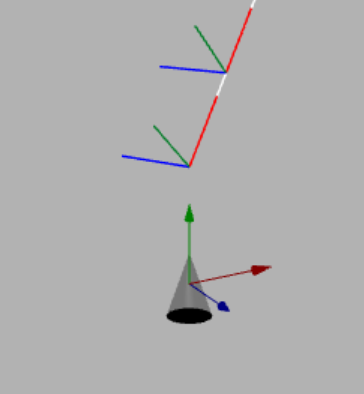# Target Axes Alignment

## Target Axes Alignment

When you want to rotate a mesh (or a camera) so that it lines up with a set of given axes you can use the RotationFromAxis method as follows

javascript
var orientation = BABYLON.Vector3.RotationFromAxis(axis1, axis2, axis3);mesh.rotation = orientation;

where axis1, axis2 and axis3 are three left-handed orthogonal vectors and the mesh will be aligned with

• axis1 as the x axis in its local space
• axis2 as the y axis in its local space
• axis3 as the z axis in its local space

## Align Plane to Curve

At any point along curve in 3D space the tangent, normal and binormal form a set of orthogonal axes, call these the point axes. A plane created in Babylon.js has a normal along the z axis with the x and y axes lying in the plane. We can draw a curve in space using an array of position vectors. By creating a 3D path from this curve we can obtain the normal, tangent and binormal of the curve at each of the positions that define it. Using the RotationFromAxis we can align the x, y and z axes of the plane with the point axes of the curve. There are 6 ways to order a group of three axes and so 6 ways to align the plane axes to the curve point axes.

All six ways are used in the playground below. The top one  has the plane tangential to the curve and the fourth one down  is perpendicular to the curve. Others can twist the plane at certain points.

Aligning a Plane To a Curve

## Refining Alignment

To help undertand which of the 6 possible arrangements it is useful to show the axes of the mesh that you want to follow the line and the tangents, normals and binormals of the path.

For example, we want a cone to follow along a path point first and with the height axes of the cone tangental to the path.

Below is an image showing the cone axes and the path tangents, normals and binormals at the start of the path before any alignment with the path takes placeOur example requires the y axis of the cone to lie along the tangents of the path. Placing the x axis of the cone along the normals of the path would leave the z axis pointing in the opposite direction to the path binormals. This gives us the the y axis along the tangents, the x axis along the binormals and the z axis along the normals. This is option 4

javascript
new BABYLON.Vector3.RotationFromAxis(binormals[p], tangents[p], normals[p]);
Aligning a Cone To a Curve

## Align Camera

Given two spheres draw a plane between them that always faces the camera. We are going to set up red and green spheres, world axes, show as red, green and blue lines, and a purple plane in the xz plane. Joining the red and green spheres is a plane with a blue arrow on it pointing from the green to the red sphere.

The requirement is for the arrow plane to rotate such that

• one of its orthogonal axes that lies on the arrow is along the line joining the two spheres;
• the other axis lying on the arrow plane is perpendicular to the camera lens;
• the axis normal to the plane is along the line joining the middle of the arrow to the camera.

In this way the camera always faces the arrow plane as can be seen in the this playground.

Aligning Camera Axes

This is achieved by forming axis 1 to join the spheres

javascript
axis1 = (sphere1.position).subtract(sphere2.position);axis3 = BABYLON.Vector3.Cross(camera.position, axis1);axis2 = BABYLON.Vector3.Cross(axis3, axis1);

axis 3 to be perpendicular axis 1 and to the axis through the camera position and axis 2 from the camera to the arrow plane.

The mesh is rotated to face the camera using

javascript
mesh.rotation = BABYLON.Vector3.RotationFromAxis(axis1, axis2, axis3);

## Using Quaternions

If you prefer using quaternions instead of Euler angles, then RotationQuaternionFromAxis() computes the required rotation quaternion to assign to the mesh property rotationQuaternion.